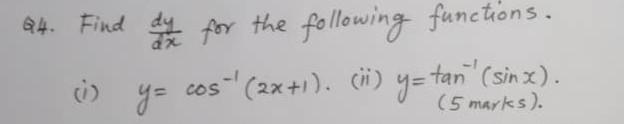### Create an Account

Already have account?

### Forgot Your Password ?

Home / Questions / 64. Find de la for the following functions. cos" (2x+1). (hi) y=tan" (sin x). y= (5 marks).

# 64. Find de la for the following functions. cos" (2x+1). (hi) y=tan" (sin x). y= (5 marks).

64. Find de la for the following functions. cos" (2x+1). (hi) y=tan" (sin x). y= (5 marks).May 09 2021 View more View Less

#### Answer (Solved)Subscribe To Get Solution